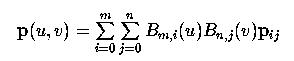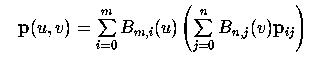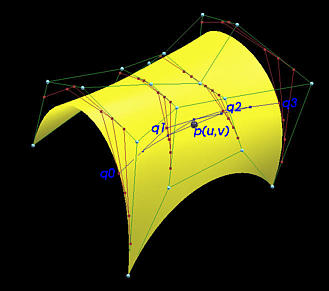# Bézier Surfaces: de Casteljau's AlgorithmDe Casteljau's algorithm can be extended to handle Bézier surfaces. More precisely, de Casteljau's algorithm can be applied several times to find the corresponding point on a Bézier surface p(u,v) given (u,v). This page describes such as extension, which is based on the concept of isoparametric curves discussed in the previous page.

Recall that the equation of a Bézier surfacecan be rewritten as the followingFor i = 0, 1, ..., m define qi(v) as follows:For a fixed v, we have m+1 points q0(v), q1(v), ..., qm(v). Each qi(v) is a point on the Bézier curve defined by control points pi0, pi1, ..., pin. Plugging these back into the surface equation yieldsThis means p(u,v) is a point of the Bézier curve defined by m+1 control points q0(v), q1(v), ..., qm(v). Thus, we have the following conclusion:

To find point p(u,v) on a Bézier surface, we can find m+1 points q0(v), q1(v), ..., qm(v) and then from these points find p(u,v).

This conclusion gives us a simple way for computing p(u,v) given (u,v). Here is why. Since each qi(v) is a point on the Bézier curve defined by the i-th row of control points: pi0, pi1, ..., pin. Therefore, for the i-th row and a given v, we can apply de Casteljau's algorithm for Bézier curve to compute qi(v). After m+1 applications of de Casteljau's algorithm (i.e., one for each row), we shall have q0(v), q1(v), ..., qm(v) in hand. Then, applying de Casteljau's algorithm to these m+1 control points again with u yields the final point p(u,v) on the surface!

The following diagram illustrates this concept. The given surface is a degree (2,2) Bézier surface defined by a 3x3 control net. Suppose u = 2/3 and v = 1/3. To determine q0(1/3), we take the 0-th row of control points p00, p01 and p02 and apply de Casteljau's algorithm to this Bézier curve with v = 1/3. Repeat this for the first row and the second row with v = 1/3. This yields three intermediate control points q0(1/3), q1(1/3) and q2(1/3). Finally, apply de Casteljau's algorithm to these three new control points with u = 2/3. The result is p(2/3,1/3) which is colored in yellow in the figure.The following is an example with the given Bézier surface displayed. The control points are shown in white. This control net has four rows and five columns and hence is a degree (3,4) Bézier surface. For each row, the intermediate polylines used for the computation of de Casteljau's algorithm is shown in red. The four intermediate control points q0, q1, q2 and q3 are shown in the figure. The intermediate polylines used for the computation of this application of de Casteljau's algorithm are shown in blue and the final point p(u,v) on the surface is shown as a red sphere.Finally, the following summarizes this algorithm:

Input: a m+1 rows and n+1 columns of control points and (u,v).
Output: point on surface p(u,v)
Algorithm:

for i := 0 to m do
begin
Apply de Casteljau's algorithm to the i-th row of control points with v;
Let the point obtained be qi(v);
end
Apply de Casteljau's algorithm to q0(v), q1(v), ..., qm(v) with u;
The point obtained is p(u,v);